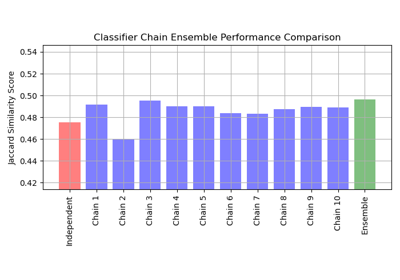# sklearn.multioutput.ClassifierChain¶

class sklearn.multioutput.ClassifierChain(base_estimator, *, order=None, cv=None, random_state=None)[source]

A multi-label model that arranges binary classifiers into a chain.

Each model makes a prediction in the order specified by the chain using all of the available features provided to the model plus the predictions of models that are earlier in the chain.

Read more in the User Guide.

New in version 0.19.

Parameters
base_estimatorestimator

The base estimator from which the classifier chain is built.

orderarray-like of shape (n_outputs,) or ‘random’, default=None

If None, the order will be determined by the order of columns in the label matrix Y.:

order = [0, 1, 2, ..., Y.shape - 1]


The order of the chain can be explicitly set by providing a list of integers. For example, for a chain of length 5.:

order = [1, 3, 2, 4, 0]


means that the first model in the chain will make predictions for column 1 in the Y matrix, the second model will make predictions for column 3, etc.

If order is ‘random’ a random ordering will be used.

cvint, cross-validation generator or an iterable, default=None

Determines whether to use cross validated predictions or true labels for the results of previous estimators in the chain. Possible inputs for cv are:

• None, to use true labels when fitting,

• integer, to specify the number of folds in a (Stratified)KFold,

• An iterable yielding (train, test) splits as arrays of indices.

random_stateint, RandomState instance or None, optional (default=None)

If order='random', determines random number generation for the chain order. In addition, it controls the random seed given at each base_estimator at each chaining iteration. Thus, it is only used when base_estimator exposes a random_state. Pass an int for reproducible output across multiple function calls. See Glossary.

Attributes
classes_list

A list of arrays of length len(estimators_) containing the class labels for each estimator in the chain.

estimators_list

A list of clones of base_estimator.

order_list

The order of labels in the classifier chain.

RegressorChain

Equivalent for regression

MultioutputClassifier

Classifies each output independently rather than chaining.

References

Jesse Read, Bernhard Pfahringer, Geoff Holmes, Eibe Frank, “Classifier Chains for Multi-label Classification”, 2009.

Examples

>>> from sklearn.datasets import make_multilabel_classification
>>> from sklearn.linear_model import LogisticRegression
>>> from sklearn.model_selection import train_test_split
>>> from sklearn.multioutput import ClassifierChain
>>> X, Y = make_multilabel_classification(
...    n_samples=12, n_classes=3, random_state=0
... )
>>> X_train, X_test, Y_train, Y_test = train_test_split(
...    X, Y, random_state=0
... )
>>> base_lr = LogisticRegression(solver='lbfgs', random_state=0)
>>> chain = ClassifierChain(base_lr, order='random', random_state=0)
>>> chain.fit(X_train, Y_train).predict(X_test)
array([[1., 1., 0.],
[1., 0., 0.],
[0., 1., 0.]])
>>> chain.predict_proba(X_test)
array([[0.8387..., 0.9431..., 0.4576...],
[0.8878..., 0.3684..., 0.2640...],
[0.0321..., 0.9935..., 0.0625...]])


Methods

 Evaluate the decision_function of the models in the chain. fit(X, Y) Fit the model to data matrix X and targets Y. get_params([deep]) Get parameters for this estimator. Predict on the data matrix X using the ClassifierChain model. Predict probability estimates. score(X, y[, sample_weight]) Return the mean accuracy on the given test data and labels. set_params(**params) Set the parameters of this estimator.
decision_function(X)[source]

Evaluate the decision_function of the models in the chain.

Parameters
Xarray-like of shape (n_samples, n_features)
Returns
Y_decisionarray-like of shape (n_samples, n_classes)

Returns the decision function of the sample for each model in the chain.

fit(X, Y)[source]

Fit the model to data matrix X and targets Y.

Parameters
X{array-like, sparse matrix} of shape (n_samples, n_features)

The input data.

Yarray-like of shape (n_samples, n_classes)

The target values.

Returns
selfobject
get_params(deep=True)[source]

Get parameters for this estimator.

Parameters
deepbool, default=True

If True, will return the parameters for this estimator and contained subobjects that are estimators.

Returns
paramsmapping of string to any

Parameter names mapped to their values.

predict(X)[source]

Predict on the data matrix X using the ClassifierChain model.

Parameters
X{array-like, sparse matrix} of shape (n_samples, n_features)

The input data.

Returns
Y_predarray-like of shape (n_samples, n_classes)

The predicted values.

predict_proba(X)[source]

Predict probability estimates.

Parameters
X{array-like, sparse matrix} of shape (n_samples, n_features)
Returns
Y_probarray-like of shape (n_samples, n_classes)
score(X, y, sample_weight=None)[source]

Return the mean accuracy on the given test data and labels.

In multi-label classification, this is the subset accuracy which is a harsh metric since you require for each sample that each label set be correctly predicted.

Parameters
Xarray-like of shape (n_samples, n_features)

Test samples.

yarray-like of shape (n_samples,) or (n_samples, n_outputs)

True labels for X.

sample_weightarray-like of shape (n_samples,), default=None

Sample weights.

Returns
scorefloat

Mean accuracy of self.predict(X) wrt. y.

set_params(**params)[source]

Set the parameters of this estimator.

The method works on simple estimators as well as on nested objects (such as pipelines). The latter have parameters of the form <component>__<parameter> so that it’s possible to update each component of a nested object.

Parameters
**paramsdict

Estimator parameters.

Returns
selfobject

Estimator instance.

## Examples using sklearn.multioutput.ClassifierChain¶